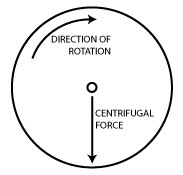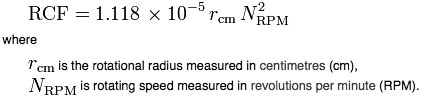# RPM Does Not Equal RCF

Posted in: Equipment Mastery and HacksRPM and RCF are two units that can be used to describe the speed of a centrifuge. Although they may look similar, they are oh-so-different and confusing them has resulted a disastrous end to many an experiment.

So let’s set it out in black and white to make sure you don’t succumb to the same mistakes as those who have passed through the labs of the world before you.

How Centrifuges WorkCentrifuges work by putting your samples in rotation around a fixed axis, thereby applying an accelerative force perpendicular to the axis as shown in the diagram on the right.

And of course, this force causes particles in your sample to accelerate toward the outer edge of the rotor. Conveniently, centrifuge manufacturers design their centrifuges so that the bottom of the sample tubes are at the outer edge of the rotor so the centrifugal force results in the sedimentation of particles in your sample to the bottom of of the tube.

The amount of force required to move a particle depends on the size of the particle. Large particles (e.g. cells) require less force than small particles (e.g. precipitated proteins). For more detailed info on this, take a look at these Wikipedia articles on sedimentation and the Lamm equation.

Describing The Force

Relative Centrifugal Force (RCF) is the term used to describe the amount of accelerative force applied to a sample in a centrifuge. RCF is measured in multiples of the standard acceleration due to gravity at the Earth’s surface (x g). This is why RCF and “x g” are used interchangeably in centrifugation protocols.

The two variables that describe RCF are the radius and the angular velocity of the rotor. i.e. how wide the rotor is and how fast it is moving.

If the rotational speed is given in revolutions per minute (RPM) and the radius is expressed in centimetres (cm), then:RCF or RPM?

From this it is clear that the correct unit for the amount of centrifugation to use in a given protocol is RCF (a.k.a. x g).

But most centrifuges, especially microcentrifuges, only have settings for RPM. So unless you are lucky enough to have a centrifuge with an RCF setting, you will have to work out the corresponding RPM that will be required for YOUR centrifuge to achieve the RCF set out in a protocol.

This can easily be done by taking your ruler, measuring the radius of your centrifuge rotor and plugging the numbers into an online converter such as this one from DJB Labcare. Alternatively, the same site also has a very useful nonograph that you can print out and keep on your bench to use for rcf to rpm conversions.

The take home message is that centrifugation speeds in quoted in RPM will only be constant for centrifuges with the same rotor radii. If you use an RPM setting from a protocol where someone used a centrifuge with a different radius from yours, you will get a different RCF. Often the difference will not be significant enough to affect the sample or the centrifuge, but sometimes, e.g. if you use an RPM setting that was originally meant for a microfuge in an ultracentrifuge, it can cause problems.

A more common mistake is to assume that RCF=RPM which can result in lab disasters, like these!

As ever, and especially since I’m a biologist pretending to be a physicist today, please feel to comment!

1.Roberto on April 16, 2010 at 3:31 pm

Hey Tess,
you can get RPM from RCF just by applying some second-grade school arithmetics to the formula provided.

RPM = [RCF / (1.118*10^-5 * r)]^0.5

e.g. for 16.000g on a 10 cm rotor,
RPM = (16000 / (1.118*10^-5 * 10)^0.5 = 11.962 RPM

You can simplify the formulae a bit of you like.
For example, with just a slight approximation,

RPM = 300 * (RCF / R)^0.5

e.g. for 16k RCF, and a 10-cm rotor,
RPM = 300 * (16000/10)^0.5 = 12.000 RPM
(a good approximation; the longer formula gives 11.963 RPM)

and

RCF = 11.2 * r * kRPM^2 (where kRPM is thousands of RPM)

e.g. for 12.000 (12k) RPM and a 10-cm rotor,
RCF = 11.2 * 10 * 12^2 = 16.128g
(a good approximation; the longer formula gives 16.099 RCF)

2.Tess on April 15, 2010 at 1:26 pm

Why don’t you write the conversion formula so that we know what to use for what.I want to convert rpm to g but everywhere people are talking about the difference.Why not put a formula

3.Jode on December 10, 2009 at 7:38 pm

The average versus maximum RCFs becomes important when there is a significant difference between the radius as measured at the top of the centrifuge bottle compared to the bottom of the bottle once mounted in the rotor. This is a reflection of the fact that the instrument spins at a particular RPM, so the actual force on the sample at the top of the bottle will be less than it is at the outside bottom corner, where the pellet will form. The Max RCF value reflects the force at the bottom corner, while average RCF is calculated using the average of the minimum and maximum radii and reflects the force exerted roughly at the center of your sample.

I think most people write their protocols using the average RCF, but this is something to consider if you are scaling a prep up. If the person who developed the protocol was using a 40ml centrifuge tube(where max and average RCF are similar), then when you scale it up to a 1L bottle (which generally has a very large sample radius difference once in the rotor) the pelleting step may not be as efficient at the same RCF, although using average RCFs attempts to mitigate these differences.

So although I think it is better to use RCFs in protocols than RPMs, you still need to have some information about what scale/rotor was being used by the protocol developer in order to make informed decisions.

4.Sean on December 9, 2009 at 5:52 am

I too would like to know about Avg vs. Max RCF values! What should I use when adopting a protocol from publications?

5.Paula on July 1, 2009 at 8:47 am

Hi Nick,
I also would like to understand the difference between RCF max and RCF avg. I need to convert settings from someone else’s protocol and would like to know if what I should use is one or the other. Can you explain? Thanks!

This site uses Akismet to reduce spam. Learn how your comment data is processed.

Scroll To Top